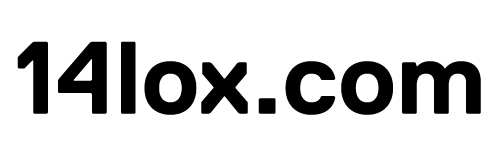#06 Aug

## drawRect() co-ordinates problem

You’ll often want to draw a rectangle using the graphics.drawRect() method, like:

```var box:Sprite = new Sprite(); box.graphics.beginFill(0x7170b6, 0.85); //drawRect(x, y, width,height) box.graphics.drawRect(100,50,300, 200); box.graphics.endFill(); addChild(box);```

where the x and y values are 100 & 50. I have found that using the x and y values inside the drawRect method to not function as I wish.
It is simply solved by setting the values outside of the method, like:

```var box:Sprite = new Sprite(); box.graphics.beginFill(0x7170b6, 0.85); box.graphics.drawRect(0,0,300, 200); box.graphics.endFill(); box.x = 100; box.y = 50; addChild(box);```